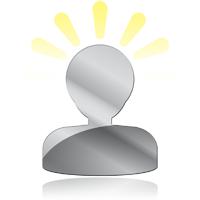## What is Binary?

Binary is the language your computer speaks. Metaphorically binary is a language of "Yes" and "No". More aptly it should be thought of as "On" or "Off". The "On" or "Off" state used in binary is represented by 1 and 0. In the language of your computer there is only 2 words, 2 numbers, 2 characters, 2 states of being, and they are 1 and 0. The reason for this is your computer uses a processor to "think". This processor has millions of transistors. Each of these transistors can either be On or Off. There is no in between.

The dictionary defines Binary as "relating to, using, or expressed in a system of numerical notation that has 2 rather than 10 as a base.". Meaning in binary there are only 2 digits instead of 10. Normally we use 1, 2, 3, 4, 5, 6, 7, 8, 9, 0 to express numbers. The 0-9 method is very useful but hard to express in computer language.

One of my favor T-Shirts says "The are only 10 types of people. Those that understand binary and those that don't.". As you can likely figure out from the second sentence in the quote the binary number "10" actually equals "2" in our standard decimal numeric system. How can this be? The following diagrams will help explain how this can be.

When you count in our standard decimal numerical system the first digit in a number represents a single quantity.

1=1, 2=2, 3=3 and so on.

The second digit represents quantities of 10.

10=10x1, 20=10x2, 30=10x3 and so on.

The third digit represents quantities of 100.

100 = 100x1
200 = 100x2
300 = 100x3 and so on.

Using this method let's express some common numbers in long form. For instance...

42 = (10x4)+2
96 = (10x9)+6
128 = (100x1)+(10x2)+8

In this decimal system each new digit is worth 10 times the digit before it. This is an example of base ten math. Binary is a base 2 math system. Thusly, in binary each new digit is worth 2 times the digit before. Let's explore this more.

Since the binary system only has 2 digits we can't represent any number higher than 1 without putting a second digit in another column. This second column however does not equal 10 like in the standard decimal numerical system, it actually equals 2. This fact is why the above quote from the aforementioned T-shirt works. Using what we just learned let's look at how 10 = 2

In binary...

0 = 1x0 = 0 (Which mathematically is 1x0, because the first column has a max value of one.)
1 = 1x1 = 1 (and that's as far as we can count without adding another digit. So let's add one.)
10 = (2x1)+0 = 2 (The 2nd digit is worth 2 times the first digit.)

With this knowledge in mind what about 3. We can now count to 3 using our two available digits and some addition...

0 = 1x0 = 0
1 = 1x1 = 1
10 = (2x1)+0 = 2
11 = (2x1)+1 = 3 (Put more simply... 2+1=3)

To better illustrate the difference between the binary system and our standard decimal numerical system we should examine the actual value of each column in these different numeric system.

### Binary System

1st Column = 0-9
2nd Column = 10
3rd Column = 100
4th Column = 1000
5th Column = 10000
6th Column = 100000
7th Column = 1000000
8th Column = 10000000
9th Column = 100000000
10th Column = 1000000000
11th Column = 100000000000
and so on...

1st Column = 1
2nd Column = 2
3rd Column = 4
4th Column = 8
5th Column = 16
6th Column = 32
7th Column = 64
8th Column = 128
9th Column = 256
10th Column = 512
11th Column = 1024
and so on...

Let's expand on this by looking at the diagram below call "Binary Column Value".Overview (Binary) 0 (Binary) 0 (Yes or No) 1 (Binary) 1 (Yes or No) 2 (Binary) 2 (Yes or No) 3 (Binary) 3 (Yes or No) 4 (Binary) 4 (Yes or No) 5 (Binary) 5 (Yes or No) 6 (Binary) 6 (Yes or No) 7 (Binary) 7 (Yes or No) 8 (Binary) 8 (Yes or No) 9 (Binary) 9 (Yes or No) 10 (Binary) 10 (Yes or No) 42 (Binary) 42 (Yes or No) 96 (Binary) 96 (Yes or No) 100 (Binary) 100 (Yes or No) 128 (Binary) 128 (Yes or No) 256 (Binary) 256 (Yes or No) 512 (Binary) 512 (Yes or No) 1024 (Binary) 1024 (Yes or No) 1100 (Binary) 1100 (Yes or No) 2011 (Binary) 2011 (Yes or No)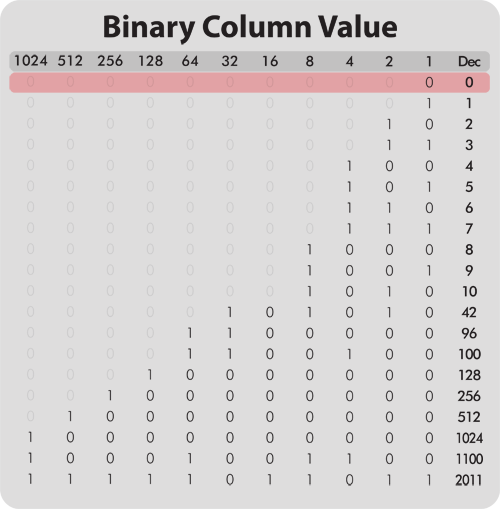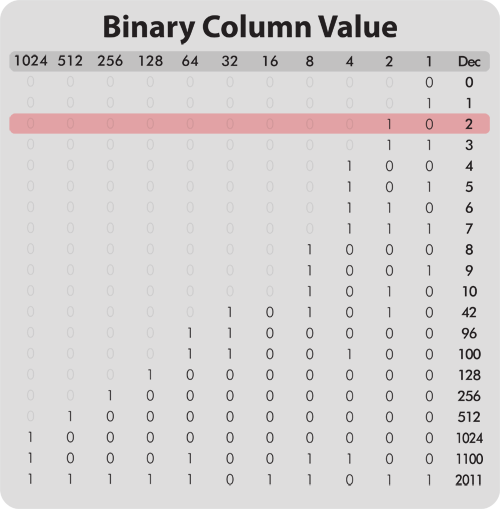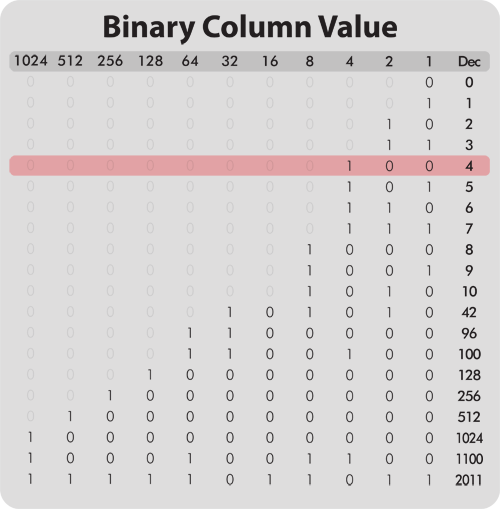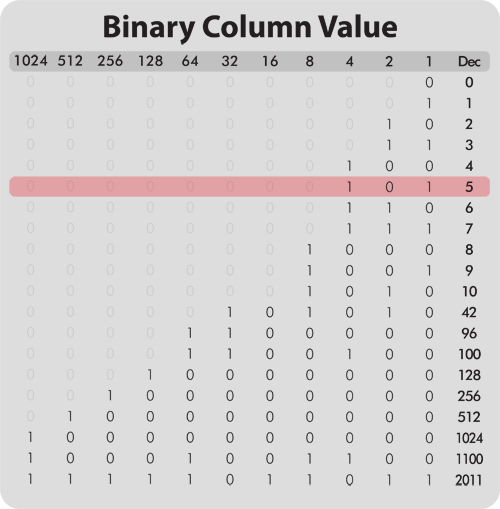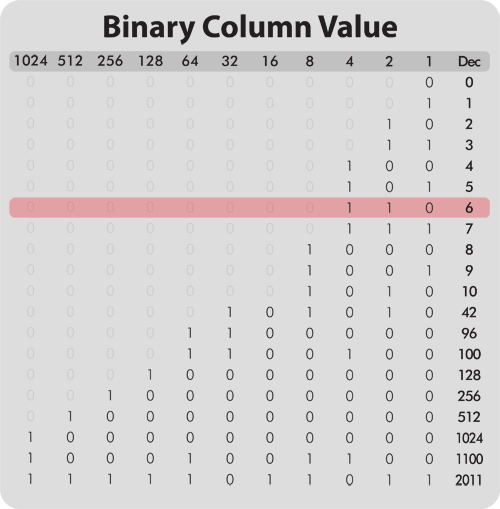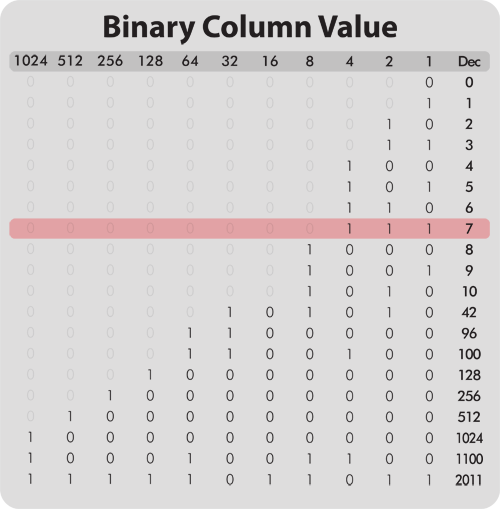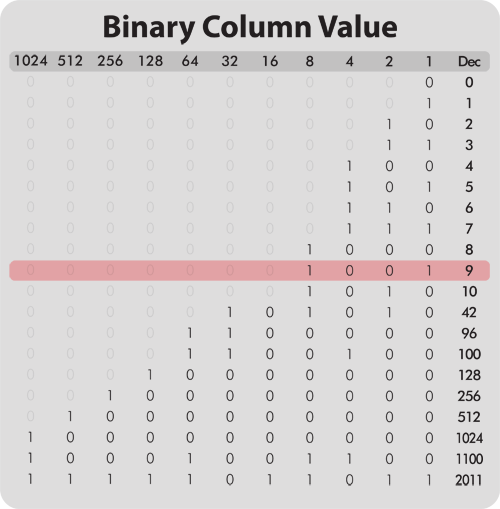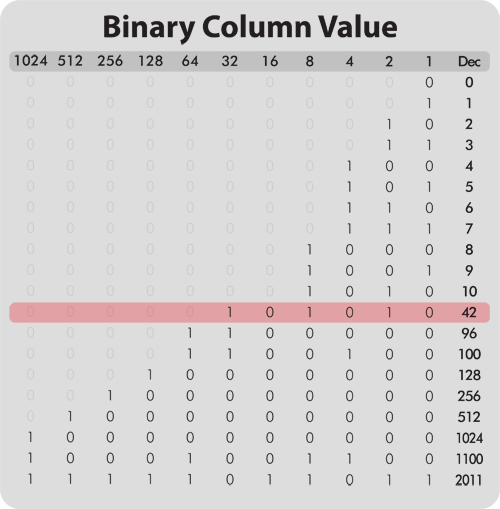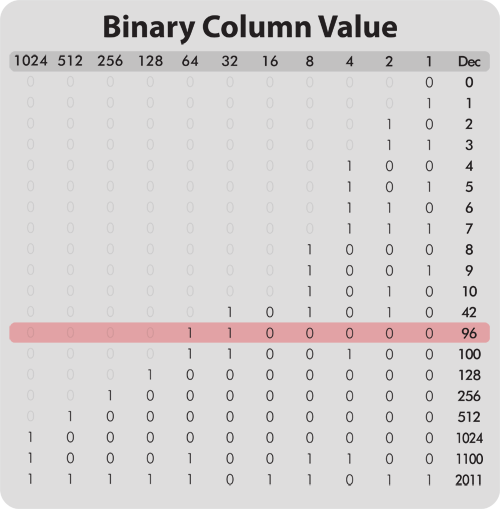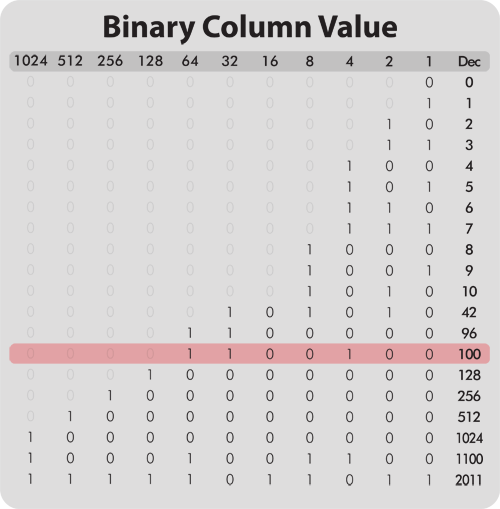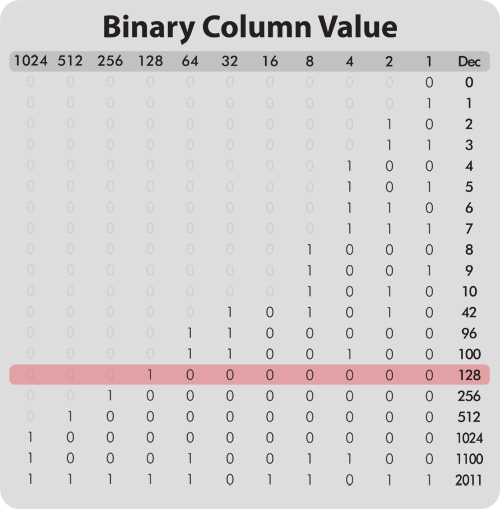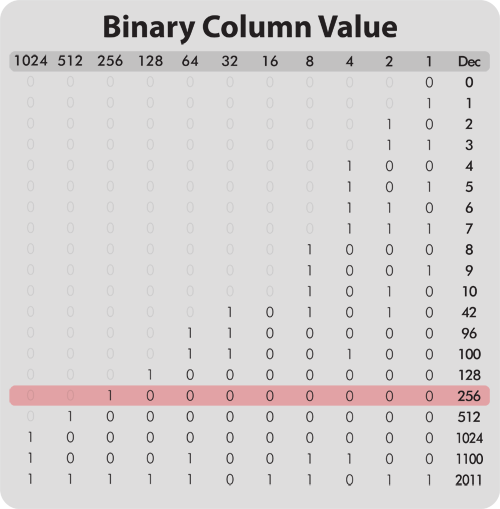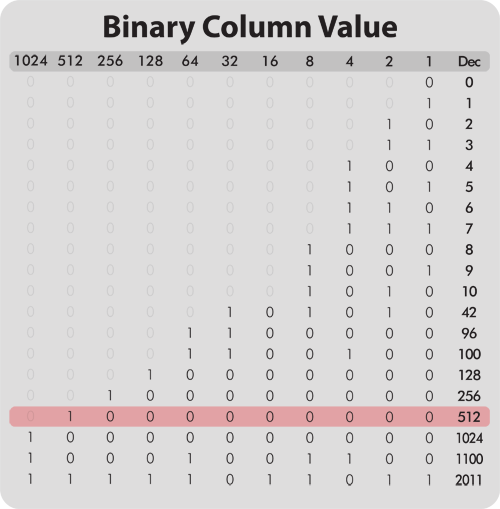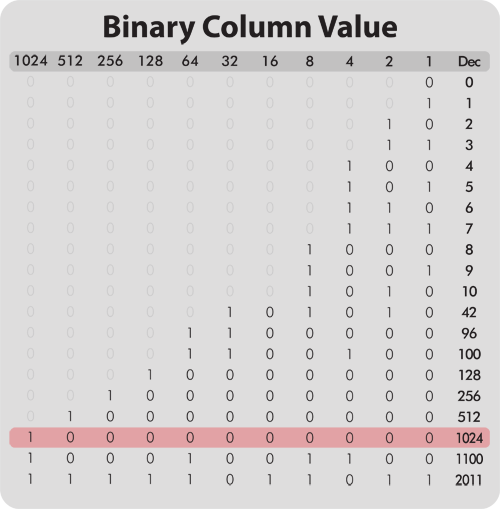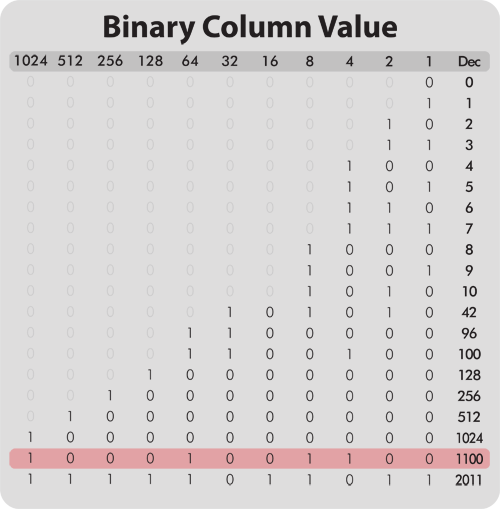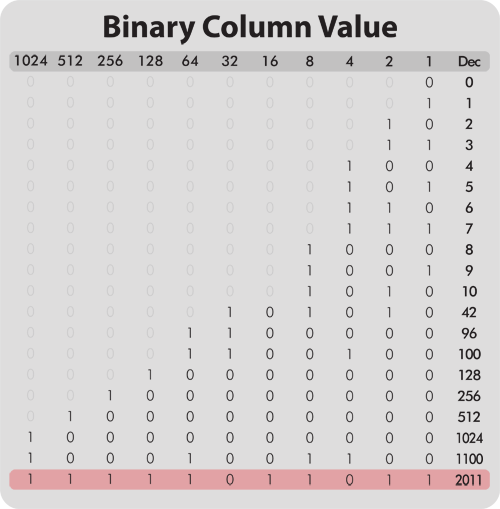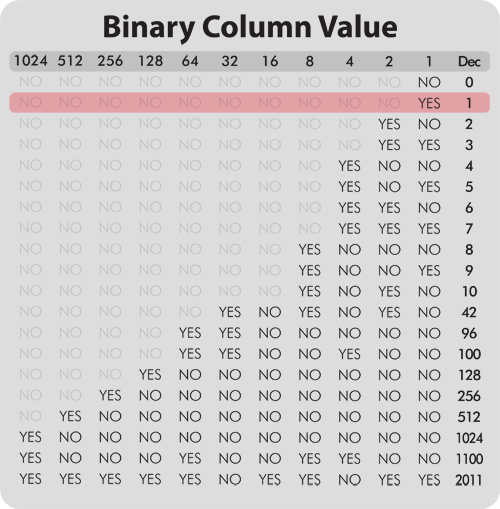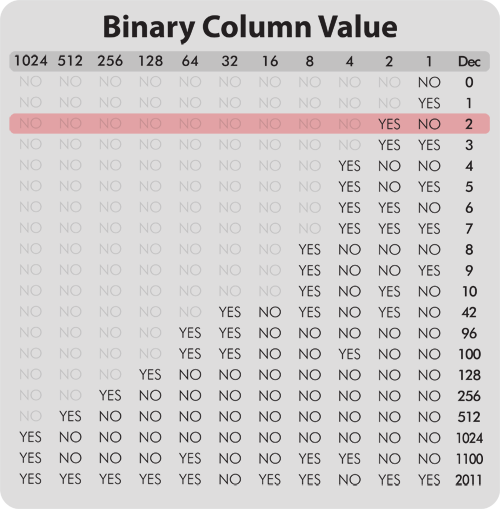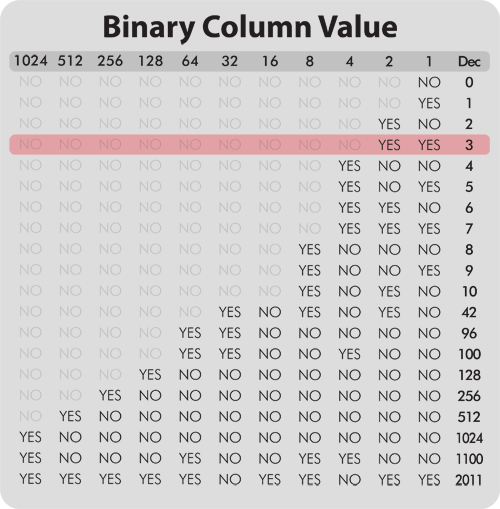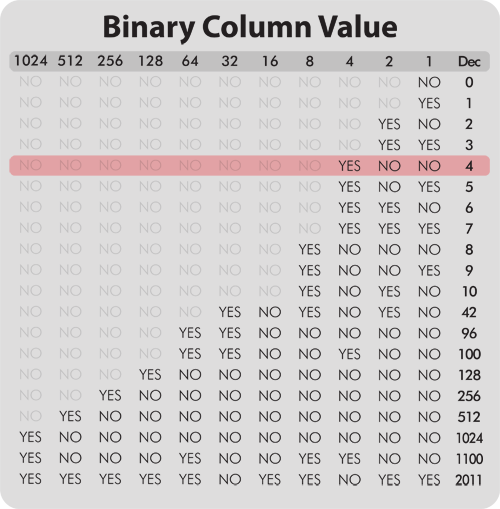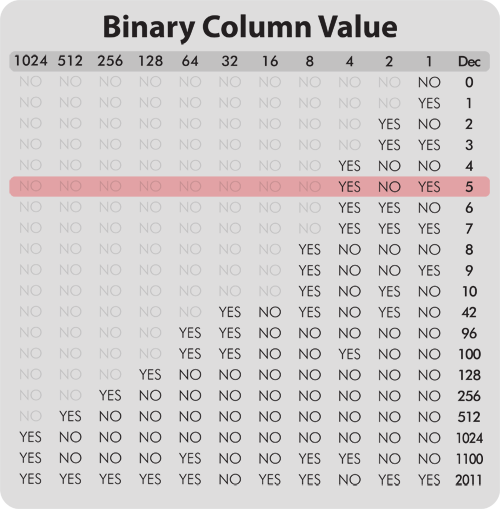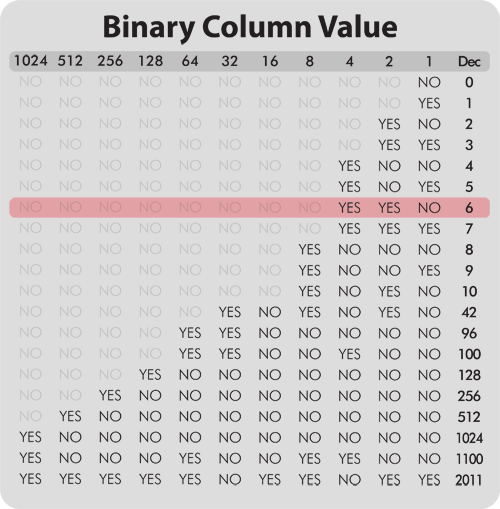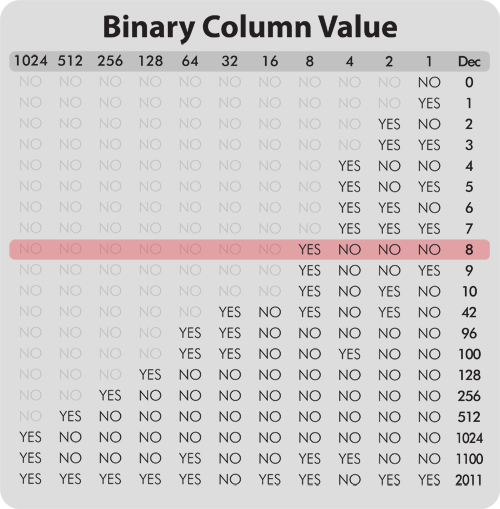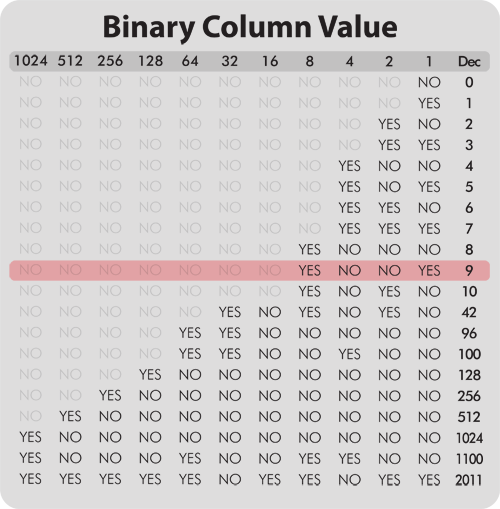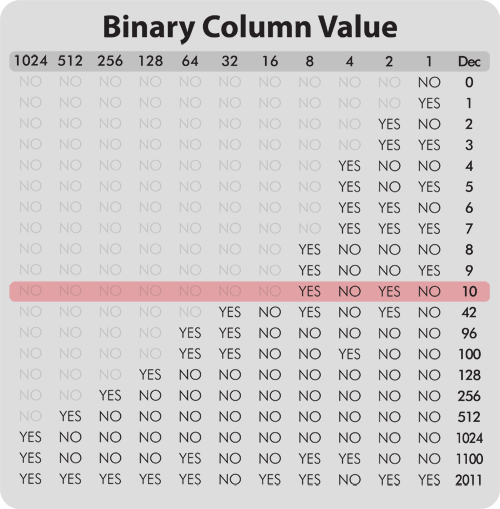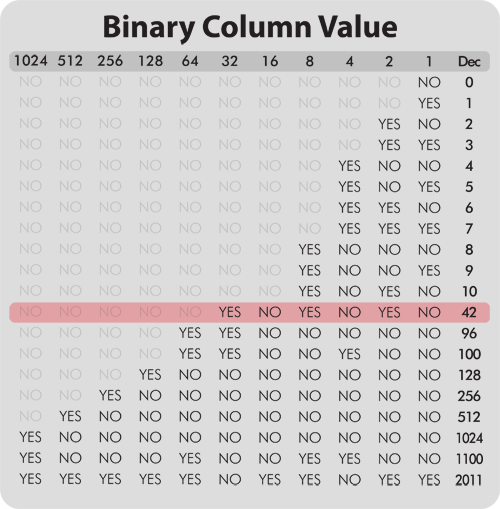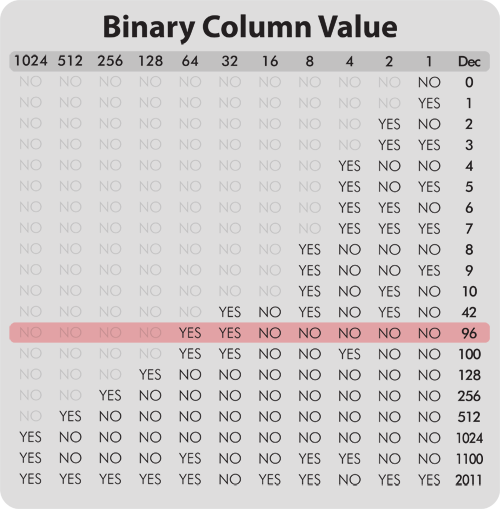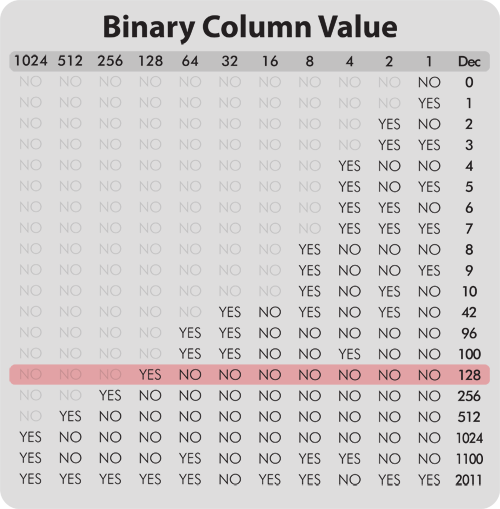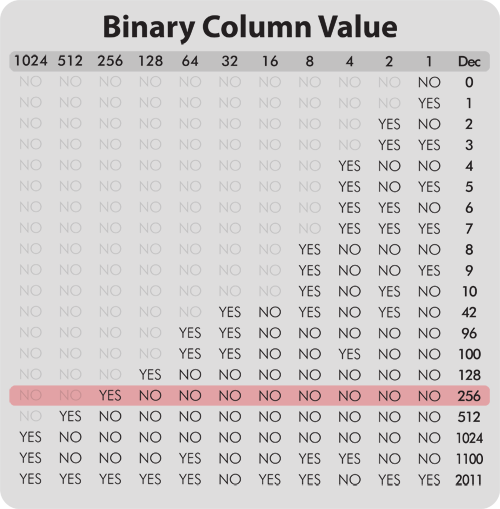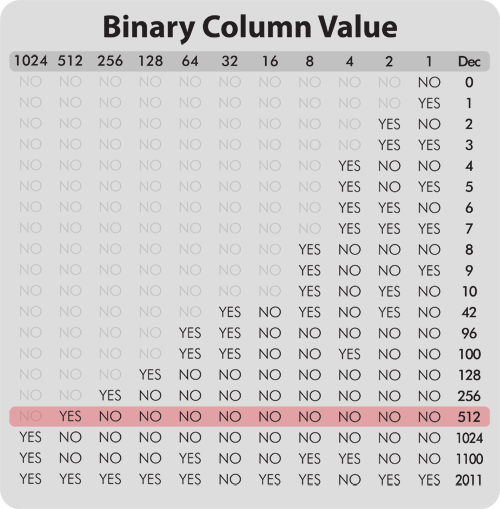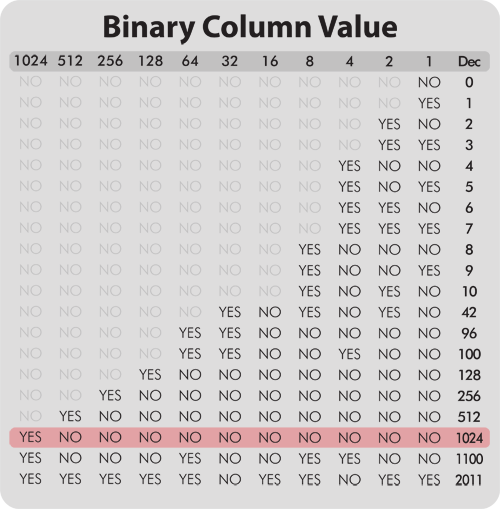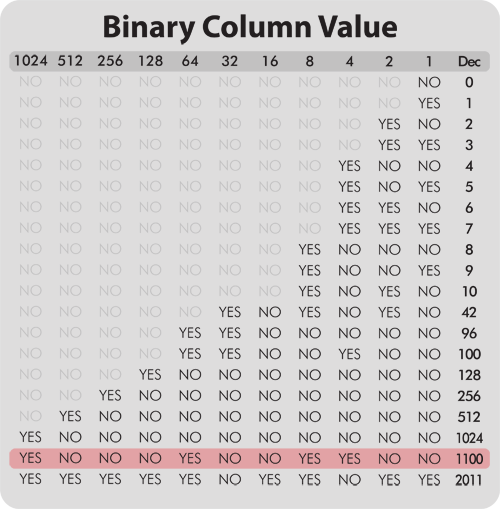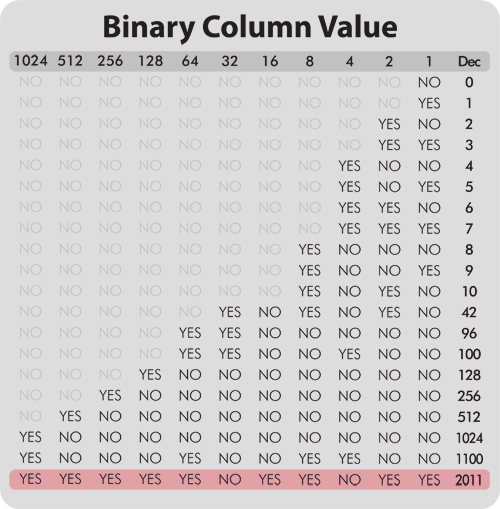As you can see at the top of this diagram, in the binary system each new column is worth 2 times the previous column instead of 10 time the previous column in the decimal system. So we either have a 1 or a 0 in the first column, in the second column we either have a 2 or a 0, in the third column we either have a 4 or a 0. Each new column doubles the value of the column before it. The far right column of this diagram shows a traditional Decimal numerical value.

With this numbering system we can represent any number possible with only 2 usable digits. The 1 or the 0.

To better understand how to figure out what a binary version of a number will be you just have to do simple subtraction. Take for instance the number 42. We know the values of each column so we just have to do some very basic math. Our number is 42. The 6th column is 32, but the 7th column is 64 so we can't use any number higher than the 6th column. So we have to start with the 6th column and start subtracting.

We take 42 and subtract 32 finding a remainder of ten. We put a 1 in the 32 column. So thus far our binary number looks like this.. 100000 because 32+0+0+0+0+0=32. Now we take the remainder and move onto the next column.

Our remainder is ten (10). Now we ask, is there 16 in 10. The answer is NO, so we move onto the next column. Is there 8 in ten (10)? Yes there is, so we put a 1 in the 8 column and have a remainder of 2. Now our binary number looks like this 101000 because 32+0+8+0+0+0=40 with a remainder of 2.

We continue onto the next column. Is there 4 in 2? No there is not so we move onto the next column. Is there 2 in 2? Yes there is, and this time we have no remainder.

So our final binary number looks like this... 101010 because 32+0+8+0+2+0=42.

You can see using this method how binary is "Yes" or "No". Do we have at least this amount? Yes or No?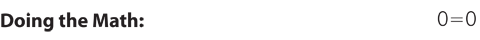0 1 2 3 4 5 6 7 8 9 10 42 96 100 128 256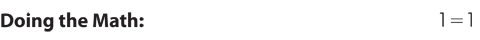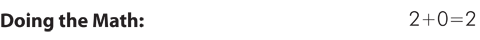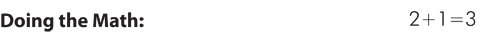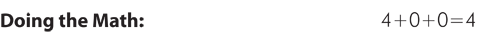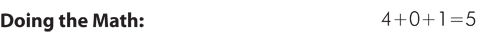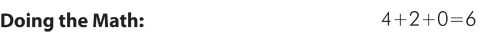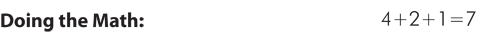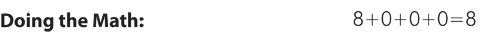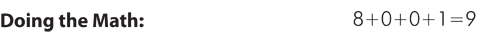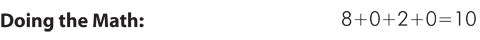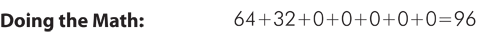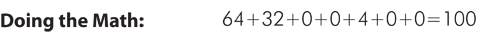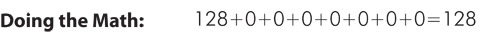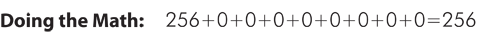So let's look at all the numbers from the list above using this subtraction / addition method. As the animation to the left scrolls through you will be able to see in decimal value addition how each number is calculated in binary. Use the forward and back arrows to move through the animation. Refer to the animation above called "Binary Column Value" for details on how each number looks in true binary form.

Using the binary system computers that have a 32 bit processor can count to 4,294,967,295 in what amounts to a single thought. Any number larger than that, a 32 bit processor has break down the number into several chucks which means larger numbers take more Processor cycles. So what is the next step in processors? 64 bit is the answer. Does that mean a 64 bit processor can count to 8,589,934,590? No, 64 bit is very much large than that. Why? Remember in binary each new digit is worth 2 times the one before it. So the 33rd bit is worth 8,589,934,590. Just adding one more 1 adds 8 and 1/2 million to our total. So what happens when we add the remaining 31 digits to get to our 64 bit goal? A very large number happens, and it is... 18,446,744,073,709,551,615.

Although understanding binary is not a prerequisite to using any computer, a basic understanding of how your computer thinks is extremely helpful when trying to grasp the underlying methodology of system design and data storage. Using nothing but 1s and 0s you computer shows you family photos, Hollywood blockbusters and allows you to write letters and emails to whom ever you want. It's amazing and true.## Contact Me

iCanHelp!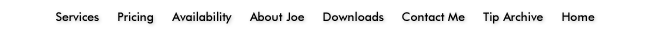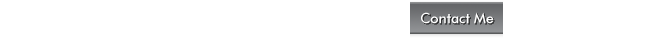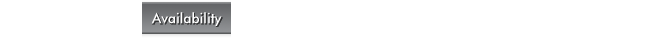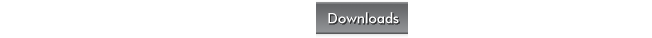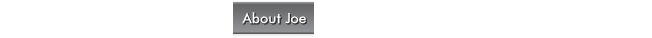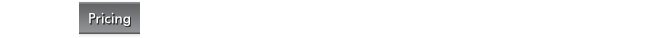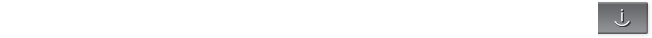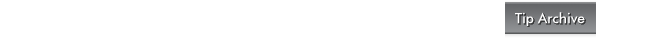iCanHelpYourMac.com© and iCanHelp!© are copyright of Joseph R Simenstad 2010. Apple Inc.©, Macintosh©, Mac© and all other copyright content owned by Apple Inc.©, are expressly owned by Apple Inc.©, and iCanHelp! does not claim any rights to any content owned expressly by Apple Inc.©.